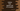# Python program to find the square root of a number## Python 3 program to find the square root of a number :

A square root of a number X is a number Y if the square of Y is equal to X or the value of Y * Y is equal to X. In this tutorial, we will learn how to find out the square root of a number in Python.

We can find out the square root of a number in python using two different approaches. In this example, we will learn both of these approaches one by one. We are using python 3 for this example.

### Required knowledge for this example :

1. if-else in python.
2. Basics of user input.

If you are learning python, and familiar with its basic concepts, you can easily grab this tutorial.

### Approaches :

We can find out the square root of a number using :

1. Simple mathematics or without using any inbuilt function.
2. Using the math module.

The source code is available here.

### Python3 Program to find square root without using the inbuilt function :

``````#1
number = int(input("Enter a number to find the square root : "))

#2
if number < 0 :
print("Please enter a valid number.")
else :
#3
sq_root = number ** 0.5
#4
print("Square root of {} is {} ".format(number,sq_root))``````

### Explanation :

The commented numbers in the above program denote the step number below:

1. First, take the input number from the user and save it in a variable number.
2. Check if the number is a negative number. We are calculating square root only for positive numbers. If it is negative, prompt the user to enter a valid number.
3. Now, to find the square root of the number, we are finding the number raised to the power 0.5. It will give us the square root of the number. Save this value in variable sq_root. e.g. if we are finding out the square root of 4, we will find 4 to the power 0.5, which is 2. Similarly, we can find out the square root of any number.
4. Finally, print out the result to the user.

### Python program to find the square root of a number using math module :

``````import math

number = int(input("Enter a number to find square root : "))

if number < 0 :
print("Please enter a valid number .")
else :
print("Square root of {} is {} ".format(number,math.sqrt(number)))``````

### Explanation :

The output of this process is the same.  We are changing the following two points here :

1. Import the math module using import math at the beginning of the program. If we use this import, we can use all methods defined inside this module. The method used to find the square root of a number is defined inside this math module.
2. Find the square root of the number using math.sqrt(number) method. This method takes one number as the parameter. It calculates the square root of that number and returns the result. We are using the format() method to print out the result to the user. This method takes two arguments: the first one is the number and the second one is the return value of the sqrt() function, i.e. the square root of the number.

You can use any of the following methods to find the square root. But always remember to check if it is a positive number or not. Else, it will throw an exception.